Op-Amp Experimentation 1: Op-Amp Basics

Op-Amp Experimentation 2: Basic Circuit Math

Op-Amp Experimentation 3: Op-Amp Applications

Op-Amp Experimentation 4: From Ideal to Real

Op-Amp Experimentation 5: Integrator

Op-Amp Experimentation 6: Differentiator

Op Amp Experimentation 7: PID Controller – coming soon
Okay, so I’m not going to post the math proving the equations, just some fun examples of what you can do with op-amps.The equation is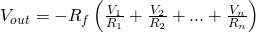and if the resistors are all equal, then it is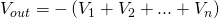.

## MultiplicationHere we have a multiplication circuit. This is actually pretty interesting, because the first two op amps take the natural log of the two inputs, then the second op amp adds them and solves the exponential of the sum. This is based on the fact that log(a*b)=log a + log b. I won’t bother posting the equation to this, because there is a much simpler way of doing things that doesn’t result in a 0.6V drop from the diodes.The equation for this is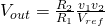## Division

This is interesting, I haven’t found any quick examples on this. However I think the easiest way would be to use a relationship similar to the one used for multiplication, although use the identity log(x/y) = log(x) – log(y). If anyone figures this out let me know, I’d love to include a picture.

## Integrator

Turns out it is pretty easy to do calculus with op-amps, as long as you understand that capacitors and inductors vary voltage/current with the rate of change of voltage/current. Here is a way to do integrationThe equation for this as long as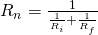is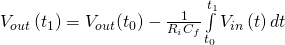## Derivative

An easy way to differentiate a signal is to use the circuit below:The equation: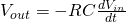## Logarithmic

We already saw an example of this in the multiplication circuit, but here it is:The equation is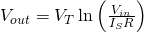Where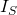is the saturation current and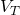is the thermal voltage of the diode.

## Exponential

Similar to the logarithmic setup, the exponential setup isAnd the equation is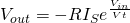## Sine Wave

Last one! To create an accurate sine wave we can use a  Wien Bridge to generate an accurate sine wave.The equation:. The component at the top is a light bulb – this is used so that it will heat up until its resistance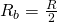. Now the resistors and capacitors do not need to equal each other, but it makes the math much simpler. I am not very familiar with these, so I’ll be sure to test it out later on.

Op-Amp Experimentation 3: Op-Amp Applications
Tagged on: